# Ex 5.1 NCERT Solutions - Introduction to Euclid’s Geometry Class 9 Notes | EduRev

## Class 9 : Ex 5.1 NCERT Solutions - Introduction to Euclid’s Geometry Class 9 Notes | EduRev

The document Ex 5.1 NCERT Solutions - Introduction to Euclid’s Geometry Class 9 Notes | EduRev is a part of the Class 9 Course Class 9 Mathematics by VP Classes.
All you need of Class 9 at this link: Class 9

Question 1. Which of the following statements are true and which are false? Give reasons for your answers.
(i) Only one line can pass through a single point.
(ii) There are an infinite number of lines which pass through two distinct points.
(iii) A terminated line can be produced indefinitely on both the sides.
(iv) If two circles are equal, then their radii are equal.
(v) In the following figures, if AB = PQ and PQ = XY, then AB = XY.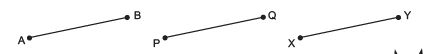Solution: (i) False

[If we mark a point O on the surface of a paper and using pencil and ruler, we can draw indefinite number of straight lines passing through O.]

(ii) False

[∵ In the following figure, there are many straight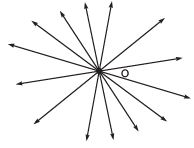lines passing through ‘P’. There are many lines, passing through ‘Q’. But there is one and only one line which is passing through ‘P’ as well as ‘Q’.]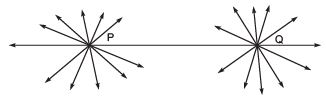(iii) True

[∵ The postulate 2 says that “A terminated line can be produced indefinitely.”]

(iv) True

[∵ Superimposing the region of one circle on the other, we find them coinciding. So, their centres and boundaries coincide. Thus, their radii will coincide.]

(v) True

[∵ According to Euclid’s axiom, things which are equal to the same thing are equal to one another.]

Question 2. Give a definition for each of the following terms. Are there other terms that need to be defined first? What are they, and how might you define them?
(i) parallel lines
(ii) perpendicular lines
(iii) line segment
(v) square
Solution:
Yes, we need to have an idea about the terms, point, line, ray, angle, plane, circle and quadrilateral, etc. before defining the required terms.
Point: A small dot made by a sharp pencil on the surface of a paper gives an idea about a point.
It has no dimensions. It has only a position.
Line: A line is an idea that it should be straight and that it should extend indefinitely in both the directions. It has no end points and has no definite length.
Note: In geometry a line means “The line in its totality and not a portion of it. Whereas a physical example of a perfect line is not possible. A line extends indefinitely in both directions, so we cannot draw or show it wholly on a paper. That is why we mark arrow heads on its both  ends, indicating that it extends indefinitely in both directions.
Ray: A part of line which has only one end point and extends indefinitely in one direction. A ray has no definite length.
Angle: Two rays having a common end-point form an angle.
Plane: Plane is a surface such that every point of the line joining any two points on it, lies on it.
Circle: A circle is the set of all those points in a given plane which are equidistant from a fixed point is the same plane. The fixed point is called the centre of the circle.
Definitions of the required terms are given below: (i) Parallel Lines: Two lines ‘l’ and ‘m’ in a plane are said to be parallel, if they have no common point and we write them as l || m.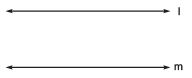Note: The distance between two parallel lines always remains the same.

(ii) Perpendicular Lines: Two lines ‘p’ and ‘q’ lying in the same plane are said to be perpendicular if they form a right angle and we write them as p ⊥ q.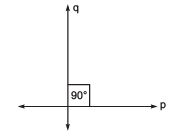(iii) Line Segment: A line segment is a part of line having a definite length. It has two end-points.
In the figure a line segment is shown having end points ‘A’ and ‘B’. It is written as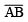or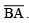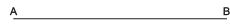(iv) Radius of a circle: The distance from the centre to a point on the circle is called the radius of the circle. In the figure, P is centre and Q is a point on the circle, then PQ is the radius.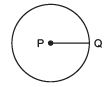(v) Square: A quadrilateral in which all the four angles are right angles and all the four sides are equal is called a square. In the figure PQRS is a square.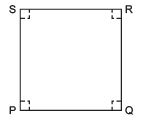Question 3. Consider two ‘postulates’ given below:
(i) Given any two distinct points A and B, there exists a third point C which is in between A and B.
(ii) There exist at least three points that are not on the same line.
Do these postulates contain any undefined terms? Are these postulates consistent?
Do they follow Euclid’s postulates? Explain.
Solution: Yes, these postulates contain undefined terms such as: Point and Line.
Also, these postulates are consistent because they deal with two different situations as (i) says that given two points ‘A’ and ‘B’. There is a point ‘C’ lying on the line in between them. Whereas (ii) says that, given ‘A’ and ‘B’, you can take ‘C’ not lying on the line through ‘A’ and ‘B’.

No, these postulates do not follow from Euclid’s postulates, however they follow from the axiom: “Given two distinct points, there is a unique line that passes through them.”

Question 4. If a point C lies between two points A and B such that AC = BC, then prove that AC =  (1/2)AB. Explain by drawing the figure.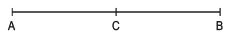∵ AC = BC      [given]
∴ AC + AC = BC + AC        [If equals added to equals then wholes are equal]
or 2AC = AB      [∵ AC + BC = AB]
or AC = (1/2)AB

Question 5. In question 4, point C is called a mid-point of the segment AB. Prove that every line segment has one and only one mid-point.
Solution: Let the given line AB is having two mid points ‘C’ and ‘D’.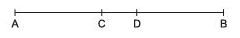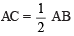...(1)
and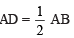...(2)
From (1) and (2), we have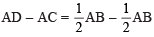or AD – AC = 0
or CD = 0
∴ C and D coincide.
Thus, every line segment has one and only one mid-point.

Question 6. In the figure, given below, if AC = BD, then prove that AB = CD.
Solution: We have: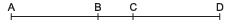AC = BD                      [Given]     ..(1)
Since, the point B lies between A and C,
∴ AC = AB + BC        ...(2)
Similarly, the point C lies between B and D,
∴ BD = CD + BC        ...(3)
From (1), (2) and (3)
AB + BC = CD + BC
[If equals are subtracted from equals, the remainders are equal]
⇒ AB = CD

Question 7. Why is Axiom 5, in the list of Euclid’s axioms, considered a ‘universal truth’? (Note that the question is not about the fifth postulate.)
Solution: In the given list of Euclid’s axioms, we have:
“The whole is greater than the part”
This statement is true for all things and in all parts of Universe.
So, it is a ‘Universal truth’.

Offer running on EduRev: Apply code STAYHOME200 to get INR 200 off on our premium plan EduRev Infinity!

132 docs

,

,

,

,

,

,

,

,

,

,

,

,

,

,

,

,

,

,

,

,

,

;